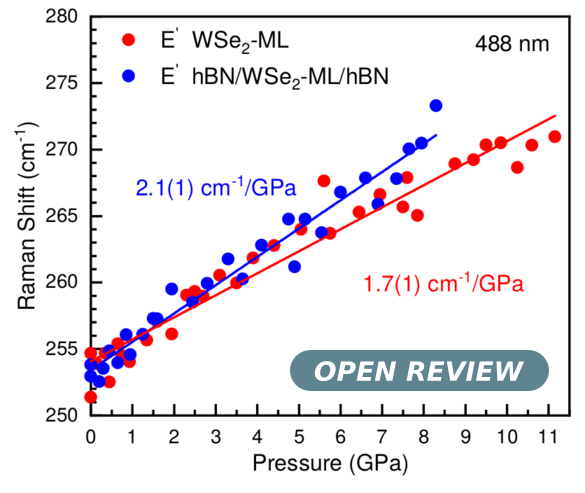# On the impact of the stress situation on the optical properties of $WSe_2$ monolayers under high pressure

## Authors

• Adrián Francisco-López Institut de Ciència de Materials de Barcelona
• Bo Han Université de Toulouse
• Delphine Lagarde Université de Toulouse
• Xavier Marie Université de Toulouse
• Bernhard Urbaszek Université de Toulouse
• Cedric Robert Université de Toulouse
• Alejandro Goñi Institut de Ciència de Materials de Barcelona

## Keywords:

dichalcogeneides, monolayers, high pressure, Raman, photoluminescence, stress-strain tensors

## Abstract

We have studied the optical properties of $WSe_2$ monolayers (ML) by means of photoluminescence (PL), PL excitation (PLE) and Raman scattering spectroscopy at room temperature and as a function of hydrostatic pressure up to ca. 12 GPa. For comparison the study comprises two cases: A single $WSe_2$ ML directly transferred onto one of the diamonds of the diamond anvil cell and a $WSe_2$ ML encapsulated into hexagonal boron nitride (hBN) layers. The pressure dependence of the A and B exciton, as determined by PL and PLE, respectively, is very different for the case of the bare $WSe_2$ ML and the $hBN/WSe_2-ML/hBN$ heterostructure. Whereas for the latter the A and B exciton energy increases linearly with increasing pressure at a rate of 3.5 to 3.8 meV/GPa, for the bare $WSe_2$ ML the A and B exciton energy decreases with a coefficient of -3.1 and -1.3 meV/GPa, respectively. We interpret that this behavior is due to a different stress situation. For a single ML the stress tensor is essentially uniaxial with the compressive stress component in the direction perpendicular to the plane of the ML. In contrast, for the substantially thicker $hBN/WSe_2-ML/hBN$ heterostructure the compression is hydrostatic. The results from an analysis of the pressure dependence of the frequency of Raman active modes comply with the interpretation of having a different stress situation in each case.

Reviewed by: A. San Miguel, Institut Lumière Matière, Université de Lyon, France; Edited by: J. S. Reparaz2019-06-21

## How to Cite

Francisco-López, A., Han, B., Lagarde, D., Marie, X., Urbaszek, B., Robert, C., & Goñi, A. (2019). On the impact of the stress situation on the optical properties of $WSe_2$ monolayers under high pressure. Papers in Physics, 11, 110005. https://doi.org/10.4279/pip.110005

Articles